Constraints in Maya direct or limit the motion of objects by connecting their attributes. You can constrain objects together so that they behave in similar ways. For instance, you can constrain the hour hand of a clock to rotate slightly whenever the minute hand moves, or you can constrain many pistons to move up and down when the first one is moved.

Constraints can also be used to ensure a certain behavior from an objecthaving eyes always point at a target or a ship always point up from the ocean, for example.

Constraints are very useful when you need to connect only certain attributes. For instance, objects can be connected in translation but rotate differently. Additionally, you can turn the constraints on and off (or partially off) as you need to, even in the middle of animation. Neither of these capabilities is available through parenting.

#### Constraint types

Maya offers the following constraint types:

• Point A point constraint connects the translate values of one object to another.

• Orient An orient constraint connects the rotate values of one object to another.

• Scale A scale constraint connects the scale values of one object to another.

• Aim An aim constraint aims one object at another object. This type of constraint can be useful for controlling where a character is looking, for example.

• Parent The parent constraint works similar to the parent function in Maya but lets you offset the child object from the parent. You can also turn the constraint on and off and choose which attributes are being affected, which you can't do with the parent function.

• Geometry A geometry constraint constrains the position of one object to the surface of another object. The pivot point of the constrained object determines where the constrained object sits on the surface.

• Normal A normal constraint constrains an object's orientation values so that they align with the normals of another surface. When used in conjunction with a geometry constraint, the normal constraint is very useful for moving characters or vehicles over bumpy surfaces.

• Tangent A tangent constraint constrains an object's orientation in the direction of a curve. If the constrained object is attached to a path, a tangent constraint ensures that the object always points in the direction of the path curve.

• Pole Vector A pole vector constraint allows you to use an object to control the pole vector value of an IK Rotate Plane handle.

#### Creating constraints

Most constraints are created in the same manner: You select the object that will control the constraint and then the object you wish to be constrained. Options such as offsets and weight give you flexibility.

To create a point constraint:

1.

Create a poly sphere and cube.

2.

Translate the cube a few units away from the sphere (Figure 10.39).

##### Figure 10.39. Constraints let you control one object with another, even if they're in different hierarchies.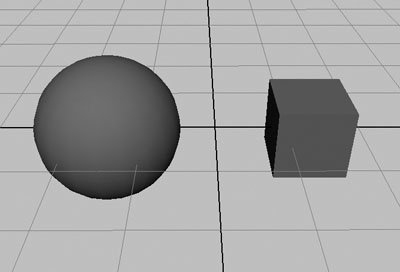3.

Select the sphere, and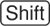-select the cube.

4.

Choose Constraint > Point.

The cube jumps so that its center point is now at the same location as the sphere's center point (Figure 10.40). This is a good way to ensure that your objects are positioned at the exact same location.

##### Figure 10.40. When Maintain Offset is deselected, a point constraint causes one object to jump to the exact location of another.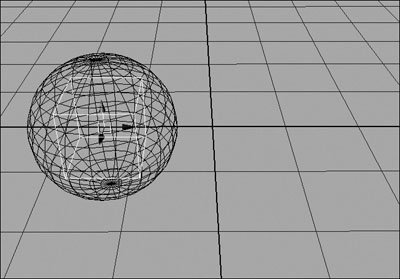5.

Undo once, so that the objects are separated again.

6.

Select the sphere, and-select the cube.

7.

From the Constraint menu, select the box next to Point.

The Point Constraint Options dialog box opens.

8.

Select Maintain Offset (Figure 10.41).

##### Figure 10.41. Maintain Offset applies the constraint relative to the object's current position, not its absolute position.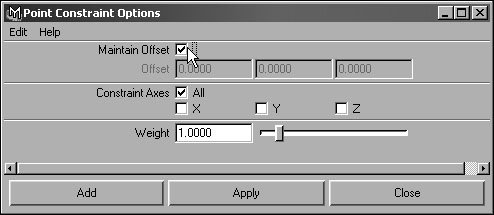This option ensures that the initial position of the cube remains the same.

9.

Click Apply.

The cube's wireframe becomes pink when the sphere is selected. This means the sphere is influencing the cube in some way.

10.

Select the sphere, and translate it in any direction using the Move tool.

The cube translates exactly the same way automatically (Figure 10.42).

##### Figure 10.42. When the sphere is moved, the cube moves as well. Because the cube's location is now defined by the sphere, it can't be moved alone.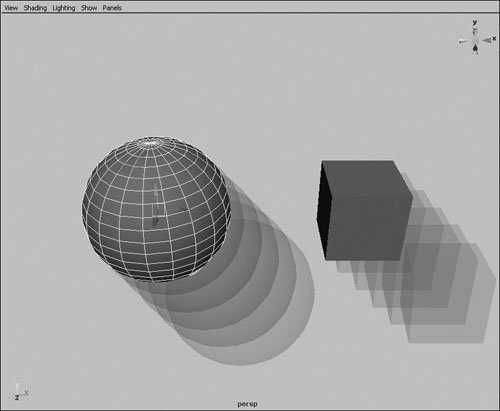Tips

• Try adding an orient constraint using the same method as described in the preceding task. Then, when you rotate the sphere, the cube will rotate in the same manner.

• The object that gets constrained is determined by which object is selected first. If you want the cube to control the sphere, you select the cube first and-select the sphere before creating the constraint.

• Constraints show up in the Outliner and Hypergraph underneath the object they're applied to (Figure 10.43). You can select this node and adjust the constraint parameters or delete it to remove the constraint.

##### Figure 10.43. You can find a constraint node under the object that is being constrained or by graphing connections in the Hypergraph.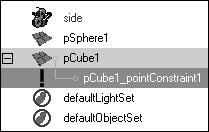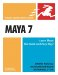Maya 7 for Windows & Macintosh
ISBN: 0321348990
EAN: 2147483647
Year: 2006
Pages: 185